# wcdiskmargin

Worst-case disk-based stability margins of uncertain feedback loops

## Syntax

``[wcDM,wcu] = wcdiskmargin(L,'siso')``
``[wcMM,wcu] = wcdiskmargin(L,'mimo')``
``[wcMMIO,wcu] = wcdiskmargin(P,C)``
``___ = wcdiskmargin(___,sigma)``
``___ = wcdiskmargin(___,opts)``
``[___,info] = wcdiskmargin(___)``

## Description

The worst-case disk margin is the smallest disk margin that occurs within a specified uncertainty range. It is also the minimum guaranteed margin over the uncertainty range. `wcdiskmargin` estimates the worst-case disk margins and corresponding worst-case gain and phase margins for both loop-at-a-time and multiloop variations. The function also returns the worst-case perturbation, the combination of uncertain elements that yields the weakest margins.

example

````[wcDM,wcu] = wcdiskmargin(L,'siso')` estimates the worst-case loop-at-a-time disk-based stability margins for the uncertain negative feedback loop `feedback(L,eye(N))`, where `N` is the number of inputs and outputs in `L`.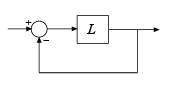While `diskmargin` computes stability margins for a nominal model, `wcdiskmargin` computes the worst-case (smallest) disk margin over the modeled uncertainty in `L`. Disk-based margin analysis provides a stronger guarantee of robust stability than the classical gain and phase margins. For general information about disk margins, see Stability Analysis Using Disk Margins.```

example

````[wcMM,wcu] = wcdiskmargin(L,'mimo')` estimates the worst-case multiloop disk margins.```

example

````[wcMMIO,wcu] = wcdiskmargin(P,C)` computes the worst-case stability margins when considering independent, concurrent variations at both the plant inputs and plant outputs the negative feedback loop of the following diagram.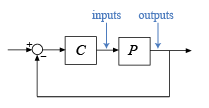```
````___ = wcdiskmargin(___,sigma)` specifies an additional skew parameter that biases the modeled gain and phase variation toward gain increase (positive `sigma`) or gain decrease (negative `sigma`). You can use this argument to test the relative sensitivity of stability margins to gain increases versus decreases. You can use this argument with any of the previous syntaxes.```
````___ = wcdiskmargin(___,opts)` specifies additional options for the computation. Use `wcOptions` to create `opts`. You can use `opts` with any of the previous syntaxes.```
````[___,info] = wcdiskmargin(___)` returns a structure with additional information about the worst-case margins and the perturbations that generate them. You can use this output argument with any of the previous syntaxes.```

## Examples

collapse all

Use `wcdiskmargin` to compute worst-case loop-at-a-time and multiloop disk margins. This example illustrates that loop-at-a-time margins can give an overly optimistic assessment of the true robustness of MIMO feedback loops. Margins of individual loops can be sensitive to small perturbations within other loops.

Consider the closed-loop system of the following illustration.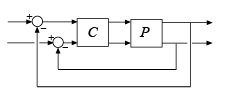P is a two-input, two-output second-order plant and C is a 2x2 static gain. Construct P in state-space form, assuming that it has an uncertain parameter and some dynamic uncertainty. Compute the worst-case disk margins at the plant output (to compute the margins at the plant input, use `L = C*Pu`).

```p = ureal('p',10,'Percentage',10); a = [-0.2 p;-p -0.2]; b = eye(2); c = [1 p;-p 1]; d = zeros(2,2); P = ss(a,b,c,0); DEL = ultidyn('DEL',[2 2],'Bound',0.1); Pu = P*(eye(2)+DEL); C = [1 -2;0 1]; L = Pu*C; [wcDM,wcu] = wcdiskmargin(L,'siso');```

Examine the worst-case loop-at-a-time disk margins, returned in the structure array `wcDM`. Each entry in this structure array contains the worst-case stability margins of the corresponding channel.

`wcDM(1) `
```ans = struct with fields: GainMargin: [0.5298 1.8875] PhaseMargin: [-34.1696 34.1696] DiskMargin: 0.6147 LowerBound: 0.6147 UpperBound: 0.6160 CriticalFrequency: 0 WorstPerturbation: [2x2 ss] ```

The result in `wcDM(1)` gives guaranteed stability margins for the specified uncertainty range. As long as the open-loop gain of the first channel changes by a factor between 0.53 and 1.88, the closed loop remains stable for all `(p,DEL)` values within the specified range. Similarly, the closed loop remains stable as long as the phase variation does not exceed 34° in absolute value.

Similarly, `wcDM(2)` shows that in the second feedback channel, the gain can vary by any factor between 0.52 and 1.93 or the phase can vary by up to 35°, and the system remains stable for such variations and the `(p,DEL)` uncertainty.

`wcDM(2)`
```ans = struct with fields: GainMargin: [0.5167 1.9352] PhaseMargin: [-35.3450 35.3450] DiskMargin: 0.6372 LowerBound: 0.6372 UpperBound: 0.6386 CriticalFrequency: 0 WorstPerturbation: [2x2 ss] ```

The lower bound returned by `wcdiskmargin` is a theoretical minimum guaranteed worst-case disk margin. The upper bound corresponds to an actual perturbation in the specified uncertainty range that approaches the lower-bound prediction. The output `wcu` contains the values of that perturbation for each feedback channel. For example, `wcu(2)` is the worst combination of `(alpha,DEL)` for the second channel, and the disk margins for this worst combination are close to `wcDM(2)`. In particular, `DM(2).UpperBound` and `wcDM(1).UpperBound` match.

```wcL = usubs(L,wcu(2)); DM = diskmargin(wcL); DM(2)```
```ans = struct with fields: GainMargin: [0.5159 1.9382] PhaseMargin: [-35.4184 35.4184] DiskMargin: 0.6386 LowerBound: 0.6386 UpperBound: 0.6386 Frequency: 0 WorstPerturbation: [2x2 ss] ```

In practice, gain and phase variations affect both channels simultaneously. To estimate the stability margins with respect to such independent and concurrent variations, examine the worst-case multiloop disk margins.

`wcMM = wcdiskmargin(L,'mimo')`
```wcMM = struct with fields: GainMargin: [0.8836 1.1317] PhaseMargin: [-7.0730 7.0730] DiskMargin: 0.1236 LowerBound: 0.1236 UpperBound: 0.1239 CriticalFrequency: 0 WorstPerturbation: [2x2 ss] ```

The multiloop margins are much weaker than when considering one loop at a time. This is because it takes a smaller amount of gain (or phase) variation to destabilize the feedback loop when both channels are subject to variations.

You can visualize how uncertainty affects the margins with `wcdiskmarginplot`. This plots the (disk-based) gain and phase margins as a function of frequency for the nominal and worst-case values of `(alpha,DEL)` as well as 20 random samples of this uncertainty. The plot shows that uncertainty weakens the margins most near DC.

```wcdiskmarginplot(L,{1e-1,1e1}) legend('location','NorthWest')```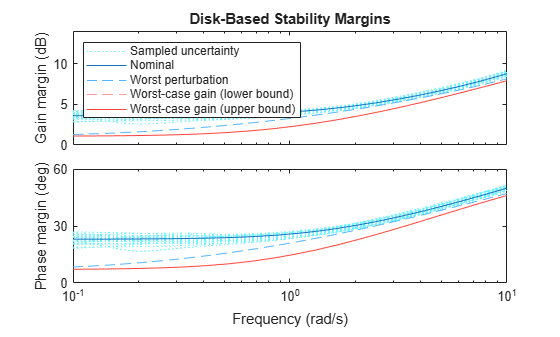Finally, compute the multiloop margin for simultaneous variations in gain (or phase) at both the plant inputs and plant outputs. When you allow the gain (or phase) to vary in more places, it becomes easier to destabilize the feedback loop, so the margins get even smaller. Thus, the multiloop I/O margin provide the most comprehensive and most conservative assessment of robust stability in the face of gain or phase variations and `(alpha,DEL)` uncertainty.

`wcMMIO = wcdiskmargin(Pu,C)`
```wcMMIO = struct with fields: GainMargin: [0.9363 1.0680] PhaseMargin: [-3.7681 3.7681] DiskMargin: 0.0658 LowerBound: 0.0658 UpperBound: 0.0659 CriticalFrequency: 1.0000e-04 WorstPerturbation: [1x1 struct] ```

## Input Arguments

collapse all

Uncertain open-loop response, specified as an uncertain model such as a `uss` or `ufrd` model. `L` can be SISO or MIMO, as long as it has the same number of inputs and outputs. `wcdiskmargin` computes the worst-case disk-based stability margins for the negative-feedback closed-loop system `feedback(L,eye(N))`.To compute the worst-case disk margins of the positive feedback system `feedback(L,eye(N),+1)`, use `wcdiskmargin(-L)`.

When you have a controller `P` and a plant `C`, you can compute the worst-case disk margins for gain (or phase) variations at the plant inputs or outputs, as in the following diagram.• To compute margins at the plant outputs, set `L = P*C`.

• To compute margins at the plant inputs, set `L = C*P`.

• To consider variations at both the plant inputs and the plant output, use the syntax `[wcMMIO,wcu] = wcdiskmargin(P,C)` instead.

`L` can be continuous time or discrete time. If `L` is a generalized state-space model (`genss`) then `wcdiskmargin` uses the current value of the tunable control design blocks in `L`.

If `L` is a frequency-response data model (such as `ufrd`), then `wcdiskmargin` computes the margins at each frequency represented in the model. The function returns the worst-case margins at the frequency with the smallest disk margin.

If `L` is a model array, then `wcdiskmargin` computes margins for each model in the array.

Plant, specified as an uncertain model such as a `uss` or `ufrd` model. `P` can be SISO or MIMO, as long as `P*C` has the same number of inputs and outputs. `wcdiskmargin` computes the worst-case disk margins for a negative-feedback closed-loop system. To compute the disk margins of the system with positive feedback, use `wcdiskmargin(P,-C)`.

`P` can be continuous time or discrete time. If `P` is a generalized state-space model (`genss`) then `wcdiskmargin` uses the current value of the tunable control design blocks in `P`.

If `P` is a frequency-response data model (such as `frd`), then `wcdiskmargin` computes the margins at each frequency represented in the model. The function returns the worst-case margins at the frequency with the smallest disk margin.

Controller, specified as a dynamic system model. `C` can be SISO or MIMO, as long as `P*C` has the same number of inputs and outputs. `wcdiskmargin` computes the disk-based stability margins for a negative-feedback closed-loop system. To compute the disk margins of the system with positive feedback, use `wcdiskmargin(-C,P)`.

`C` can be continuous time or discrete time. If `C` is a generalized state-space model (`genss`) then `wcdiskmargin` uses the current value of the tunable control design blocks in `C`.

If `C` is a frequency-response data model (such as `frd`), then `wcdiskmargin` computes the margins at each frequency represented in the model. The function returns the worst-case margins at the frequency with the smallest disk margin.

Skew of uncertainty region used to compute the stability margins, specified as a real scalar value. This parameter biases the uncertainty used to model gain and phase variations toward gain increase or gain decrease.

• The default `sigma` = 0 uses a balanced model of gain variation in a range `[gmin,gmax]`, with ```gmin = 1/gmax```.

• Positive `sigma` uses a model with more gain increase than decrease (`gmin > 1/gmax`).

• Negative `sigma` uses a model with more gain decrease than increase (`gmin < 1/gmax`).

Use the default `sigma` = 0 to get unbiased estimates of gain and phase margins. You can test relative sensitivity to gain increase and decrease by comparing the margins obtained with both positive and negative `sigma` values. For more detailed information about how the choice of `sigma` affects the margin computation, see Stability Analysis Using Disk Margins.

Options for worst-case computation, specified as an object you create with `wcOptions`. The available options include settings that let you:

• Extract frequency-dependent worst-case margins.

• Examine the sensitivity of the worst-case margins to each uncertain element.

• Improve the results of the worst-case margin calculation by setting certain options for the underlying `mussv` calculation.

For more information about all available options, see `wcOptions`.

Example: `wcOptions('Sensitivity','on','MussvOptions','m3')`

## Output Arguments

collapse all

Worst-case disk margins for each feedback channel with all other loops closed, returned as a structure for SISO feedback loops, or an N-by-1 structure array for a MIMO loop with N feedback channels. The fields of `wcDM(i)` are:

FieldValue
`GainMargin`Minimum guaranteed disk-based gain margin of the corresponding feedback channel, returned as a vector of the form `[gmin,gmax]`. These values mean that as long as the open-loop gain of the ith channel changes by a factor no less than `gmin` and no more than `gmax`, the closed loop remains stable for all uncertainty values within the ranges specified in `L`. If the open-loop gain can change sign without loss of stability, `gmin` can be less than zero for large enough negative `sigma`. If the closed-loop system goes unstable for some combination of uncertainty values, then `wcDM(i).GainMargin = [1 1]`.
`PhaseMargin`Minimum guaranteed disk-based phase margin of the corresponding feedback channel, returned as a vector of the form `[-pm,pm]` in degrees. If the closed-loop system goes unstable for some combination of uncertainty values, then `wcDM(i).PhaseMargin = [0 0]`.
`DiskMargin`Minimum guaranteed disk margin (see Stability Analysis Using Disk Margins for the definition and interpretation of the disk margin). If the closed-loop system is unstable for some combination of uncertain-element values, then `wcDM(i).DiskMargin = 0`.
`LowerBound`Lower bound on worst-case disk margin. This value is the same as `DiskMargin`.
`UpperBound`Upper bound on worst-case disk margin. This value is the disk margin obtained for the worst perturbation found by `wcdiskmargin`, returned as `wcu``(i)`. The actual worst-case disk margin is no better than this value.
`CriticalFrequency`Frequency at which the disk margin for the worst perturbation `wcu(i)` is weakest, as a function of frequency. This value is in rad/`TimeUnit`, where `TimeUnit` is the `TimeUnit` property of `L`.
`WorstPerturbation`

Smallest gain and phase variation that drives the feedback loop unstable for the worst-case combination of uncertain elements. The perturbation is returned as a state-space (`ss`) model with N inputs and outputs, where N is the number of inputs and outputs in `L`. The system `F(s) = WorstPerturbation` is such that for the worst-case combination of uncertain elements of `L` (the values returned in `wcu`) the following feedback loop has a pole on the stability boundary at `wcDM(i).CriticalFrequency`.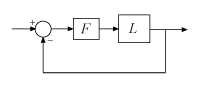This state-space model is a diagonal perturbation of the form `F(s) = diag(f1(s),...,fN(s))`. Each `fj(s)` is a real-parameter dynamic system that realizes the worst-case complex gain and phase variation applied to each channel of the feedback loop. For the loop-at-a-time margin of the kth feedback loop, only the kth entry `fk(s)` of `wcDM(k).WorstPerturbation` differs from unity.

For more information on interpreting `wcDM(K).WorstPerturbation`, see Disk Margin and Smallest Destabilizing Perturbation

This field is different from the `WorstPerturbation` field of the `info` output argument. That field contains the values of the uncertain elements of `L` that yield the smallest margins at each frequency.

When `L = P*C` is the open-loop response of a system comprising a controller and plant with unit negative feedback in each channel, `wcDM` contains the stability margins for variations at the plant outputs. To compute the stability margins for variations at the plant inputs, use `L = C*P`. To compute the stability margins for simultaneous, independent variations at both the plant inputs and outputs, use ```wcMMIO = wcdiskmargin(P,C)```.

When `L` is a model array, `wcDM` has additional dimensions corresponding to the array dimensions of `L`. For instance, if `L` is a 1-by-3 array of two-input, two-output models, then `wcDM` is a 2-by-3 structure array. `wcDM(j,k)` contains the margins for the jth feedback channel of the kth model in the array.

Perturbation of uncertain elements yielding the weakest margins, returned as:

• A structure array of dimensions N-by-1 for loop-at-a-time margins, where N is the number of feedback channels

• A scalar structure for multiloop margins

The lower bound returned by `wcdiskmargin` is a theoretical minimum guaranteed worst-case disk margin. The upper bound corresponds to an actual perturbation in the specified uncertainty range that approaches the lower-bound prediction. `wcu` contains the values of that perturbation. For example, if the input system includes uncertain elements `M` and `delta`, then `wcu.M` and `wcu.delta` contain the worst perturbations found by `wcdiskmargin`. It is possible that a worse perturbation exists, but no perturbation can yield a worse margin than the lower bound returned by `wcdiskmargin`.

Use `usubs` to substitute these values for the uncertain elements in the input system, to obtain the dynamic system that has the worst-case disk margin.

Worst-case multiloop disk margins, returned as a structure. The gain (or phase) margins quantify how much gain variation (or phase variation) the system can tolerate in all feedback channels at once while remaining stable. Thus, `wcMM` is a single structure regardless of the number of feedback channels in the system. (For SISO systems, `wcMM` = `wcDM`.) The fields of `wcMM` are:

FieldValue
`GainMargin`Minimum guaranteed multiloop disk-based gain margin, returned as a vector of the form `[gmin,gmax]`. These values mean that as long as the gain in all loop channels changes by a factor no less than `gmin` and no more than `gmax`, the closed loop remains stable for all uncertainty values within the ranges specified in `L`. If the closed-loop system goes unstable for some combination of uncertainty values, then ```wcMM.GainMargin = [1 1]```.
`PhaseMargin`Minimum guaranteed multiloop disk-based phase margin, returned as a vector of the form `[-pm,pm]` in degrees. If the closed-loop system goes unstable for some combination of uncertainty values, then `wcMM.PhaseMargin = [0 0]`.
`DiskMargin`Minimum guaranteed disk margin (see Stability Analysis Using Disk Margins for the definition and interpretation of the disk margin). If the closed-loop system is unstable for some combination of uncertain-element values, then `wcMM.DiskMargin = 0`.
`LowerBound`Lower bound on worst-case disk margin. This value is the same as `DiskMargin`.
`UpperBound`Upper bound on worst-case disk margin. This value is the disk margin obtained for the worst perturbation found by `wcdiskmargin`, returned as `wcu`. The actual worst-case multiloop disk margin is no better than this value.
`CriticalFrequency`Frequency at which the disk margin for the worst perturbation `wcu` is weakest, as a function of frequency. This value is in rad/`TimeUnit`, where `TimeUnit` is the `TimeUnit` property of `L`.
`WorstPerturbation`

Smallest gain and phase variation that drives the feedback loop unstable for the worst-case combination of uncertain elements. The perturbation is returned as a state-space (`ss`) model with N inputs and outputs, where N is the number of inputs and outputs in `L`. The system `F(s) = WorstPerturbation` is such that for the worst-case combination of uncertain elements of `L` (the values returned in `wcu`) the following feedback loop has a pole on the stability boundary at `wcMM.CriticalFrequency`.This state-space model is a diagonal perturbation of the form `F(s) = diag(f1(s),...,fN(s))`. Each `fj(s)` is a real-parameter dynamic system that realizes the worst-case complex gain and phase variation applied to each channel of the feedback loop.

For more information on interpreting `wcDM(K).WorstPerturbation`, see Disk Margin and Smallest Destabilizing Perturbation

This field is different from the `WorstPerturbation` field of the `info` output argument. That field contains the values of the uncertain elements of `L` that yield the smallest margins at each frequency.

When `L = P*C` is the open-loop response of a system comprising a controller and plant with unit negative feedback in each channel, `wcMM` contains the stability margins for variations at the plant outputs. To compute the stability margins for variations at the plant inputs, use `L = C*P`. To compute the stability margins for simultaneous, independent variations at both the plant inputs and outputs, use ```wcMMIO = wcdiskmargin(P,C)```.

When `L` is a model array, `wcMM` is a structure array with one entry for each model in `L`.

Worst-case disk margins for independent variations in all input and output channels of the plant `P`, returned as a structure having the same fields as `wcMM`.

For variations applied simultaneously at inputs and outputs, the `WorstPerturbation` field is itself a structure with fields `Input` and `Output`. Each of these fields contains a state-space model such that for ```Fi(s) = wcMMIO.WorstPerturbation.Input``` and ```Fo(s) = wcMMIO.WorstPerturbation.Output```, the system of the following diagram is marginally unstable, with a pole on the stability boundary at the frequency `wcMMIO.CriticalFrequency`, when `P` is evaluated with the worst-case uncertainty values `wcu`.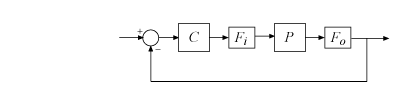These state-space models `Input` and `Output` are diagonal perturbations of the form `F(s) = diag(f1(s),...,fN(s))`. Each `fj(s)` is a real-parameter dynamic system that realizes the worst-case complex gain and phase variation applied to each channel of the feedback loop.

Additional information about the worst-case values, returned as a structure with the following fields:

FieldDescription

`Model`

Index of the model that has the smallest disk margin, when `L` is an array of models.

`Frequency`

Frequency points at which `wcdiskmargin` returns the minimum guaranteed margins, returned as a vector.

• If the `'VaryFrequency'` option of `wcOptions` is `'off'`, then `info.Frequency` is the critical frequency, the frequency at which the worst-case disk margin occurs. If the largest lower bound and the smallest upper bound on the worst-case disk margin occur at different frequencies, then `info.Frequency` is a vector containing these two frequencies.

• If the `'VaryFrequency'` option of `wcOptions` is `'on'`, then `info.Frequency` contains the frequencies selected by `wcdiskmargin`. These frequencies are guaranteed to include the frequency at which the worst-case disk margin occurs.

The `'VaryFrequency'` option is meaningful only for `uss` and `genss` models. `wcdiskmargin` ignores the option for `ufrd` and `genfrd` models.

`Bounds`

Lower and upper bounds on the actual worst-case disk margin of the model, returned as an array. `info.Bounds(:,1)` contains the lower bound at each corresponding frequency in `info.Frequency`, and `info.Bounds(:,2)` contains the corresponding upper bounds.

`WorstPerturbation`

Worst perturbations at each frequency point in `info.Frequency`, returned as a structure array. Here, worst refers to the perturbations that cause the smallest disk margin at a particular frequency. The fields of `info.WorstPerturbation` are the names of the uncertain elements in the input model. Each field contains the worst value of the corresponding element at each frequency. For example, if `L` includes an uncertain parameter `p` and SISO uncertain dynamics `delta`, then `info.WorstPerturbation.p` is a collection of numeric values and `info.WorstPerturbation.delta` is a collection of SISO state-space models.

`info.WorstPerturbation` contains the minimum-margin values of the uncertain elements in the input system `L` or `P`. It is distinct from the `WorstPerturbation` field of the output structures `wcDM`, `wcMM`, and `wcMMIO`. Those field contain state-space models representing the smallest gain and phase variations that drive the feedback loop unstable.

`Sensitivity`

Sensitivity of the worst-case disk margin to each uncertain element, returned as a structure when the `'Sensitivity'` option of `wcOptions` is `'on'`. The fields of `info.Sensitivity` are the names of the uncertain elements in the input model. Each field contains a percentage that measures how much the uncertainty in the corresponding element affects the worst disk margin. For example, if `info.Sensitivity.p` is 50, then a given fractional change in the uncertainty range of `p` causes half as much fractional change in the worst disk margin.

If the `'Sensitivity'` option of `wcOptions` is off (the default setting), then `info.Sensitivity` is `NaN`.

## Tips

• `wcdiskmargin` assumes negative feedback. To compute the worst-case disk margins of a positive feedback system, use `wcdiskmargin(-L)` or `wcdiskmargin(P,-C)`.

• You can visualize worst-case disk margins with `wcdiskmarginplot`.

## Algorithms

`wcdiskmargin` models gain (and phase) variation as `umargin` uncertainty, combines it with the specified plant uncertainty, and uses `mussv` to compute the worst-case disk margins and perturbation. This generalizes the `diskmargin` algorithm to feedback loops with uncertainty. For more information about disk-margin computation and interpretation, see Stability Analysis Using Disk Margins.

## Compatibility Considerations

expand all

Behavior changed in R2020a

### Topics

Introduced in R2018b

## SupportGet trial now